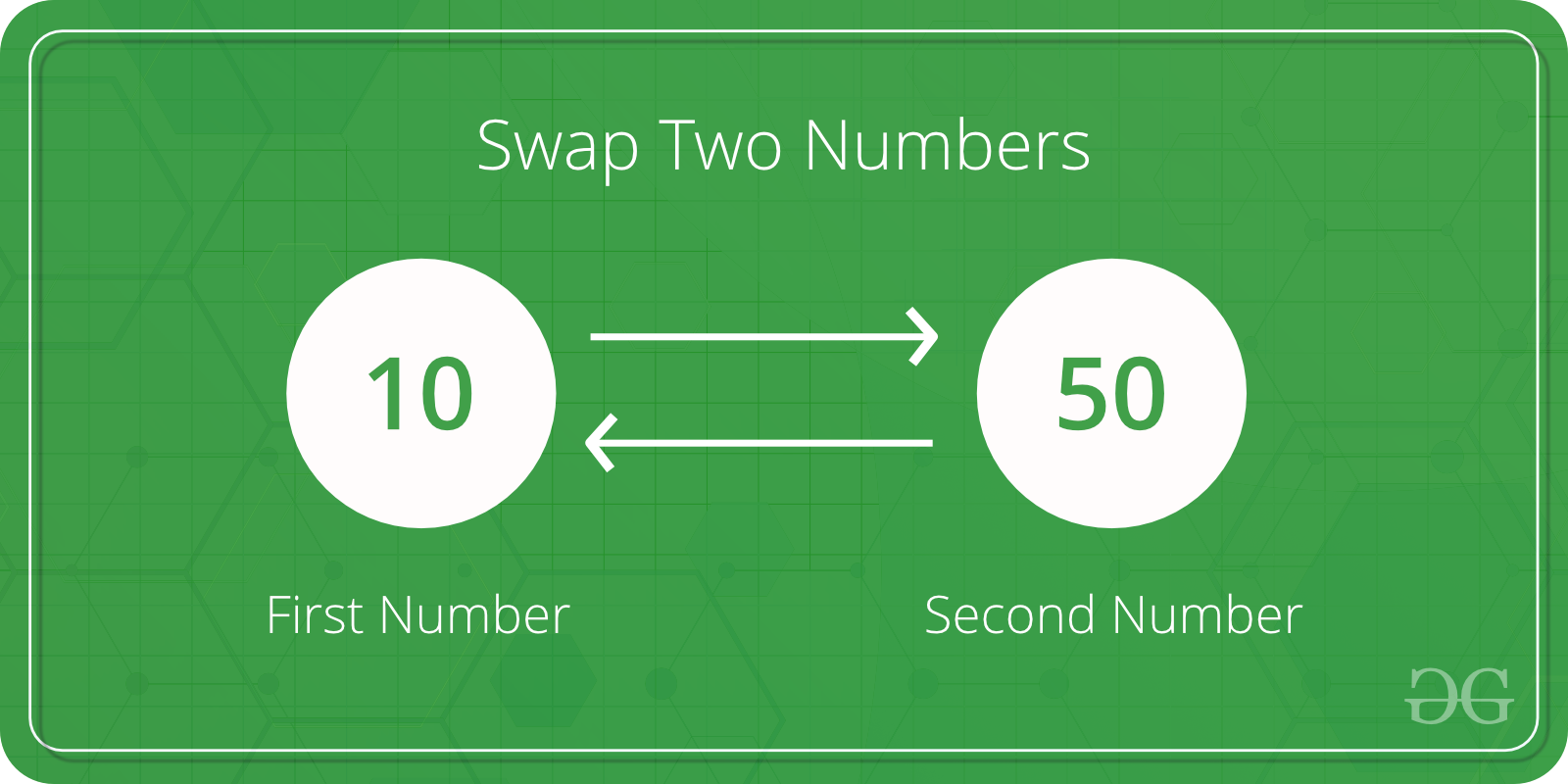# Java Program to Swap two Variables

Given two numbers x and y, we need to swap their values

Examples:

```Input : x = 10, y = 20;
Output : x = 20, y = 10

Input : x = 200, y = 100
Output : x = 100, y = 200
```Below are the simple steps we follow:
1) Assign x to a temp variable : temp = x
2) Assign y to x : x = y
3) Asign temp to y : y = temp

Let us understand with an example.

x = 100, y = 200

After line 1: temp = x
temp = 100

After line 2: x = y
x = 200

After line 3 : y = temp
y = 100

 `// Java program to swap two variables ` `public` `class` `GfG { ` ` `  `    ``public` `static` `void` `main(String[] args) ` `    ``{ ` ` `  `        ``int` `x = ``100``, y = ``200``; ` ` `  `        ``System.out.println(``"Before Swap"``); ` `        ``System.out.println(``"x = "` `+ x); ` `        ``System.out.println(``"y = "` `+ y); ` ` `  `        ``int` `temp = x; ` `        ``x = y; ` `        ``y = temp; ` ` `  `        ``System.out.println(``"After swap"``); ` `        ``System.out.println(``"x = "` `+ x); ` `        ``System.out.println(``"y = "` `+ y); ` `    ``} ` `} `

Output:

```Before Swap
x = 100
y = 200
After swap
x = 200
y = 100
```
My Personal Notes arrow_drop_upCheck out this Author's contributed articles.

If you like GeeksforGeeks and would like to contribute, you can also write an article using contribute.geeksforgeeks.org or mail your article to contribute@geeksforgeeks.org. See your article appearing on the GeeksforGeeks main page and help other Geeks.

Please Improve this article if you find anything incorrect by clicking on the "Improve Article" button below.

Article Tags :

1

Please write to us at contribute@geeksforgeeks.org to report any issue with the above content.